Join one of our upcoming courses taking place around the world! Explore now

# 8 - Automate Probabilistic Analysis

## 1.0 Introduction

The Automate Compute option helps users quickly generate results for several RocTopple analysis cases at a time by reading in various model inputs from Excel. In this tutorial, we will familiarize you with the Automate Compute feature by automatting the computation of 3 Probabilistic cases, each case with 5,000 samples (i.e., 3 x 5,000 = 15,000 toppling cases).

Topics Covered in this Tutorial:

• Probabilistic Analysis RocTopple Model
• Input Excel File Format
• Adding Input Excel File(s) to Automate Compute File Queue
• Output RocTopple Automate Compute Results File
• RocTopple Automate Compute Error Log

Finished Product:

The finished products of this tutorial can be found in the Tutorial 8 Automate Compute - Probabilistic Analysis folder, located in the Examples > Tutorials folder in your RocTopple installation folder. Inside, you will find:

• Tutorial 08 Automate Compute - Probabilistic_base file.rtop RocTopple model file setup for automation
• Tutorial 08 Automate Compute - Probabilistic_inputs.xlsx Excel input file
• Tutorial 08 Automate Compute - Probabilistic_inputs folder containing the computed results files
• Tutorial 08 Automate Compute - Probabilistic_post-process.xlsx Excel file with all raw combined results data, Excel formula computations, and Excel graph

## 2.0 Creating a New File

1. If you have not already done so, run the RocTopple program by double-clicking the RocTopple icon in your installation folder or by selecting Programs > Rocscience > RocTopple > RocTopple in the Windows Start menu.

When the program starts, a default model is automatically created. If you do NOT see a model on your screen:

1. Select: File > New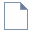Whenever a new file is created, the default input data forms a valid wedge, as shown in the image below.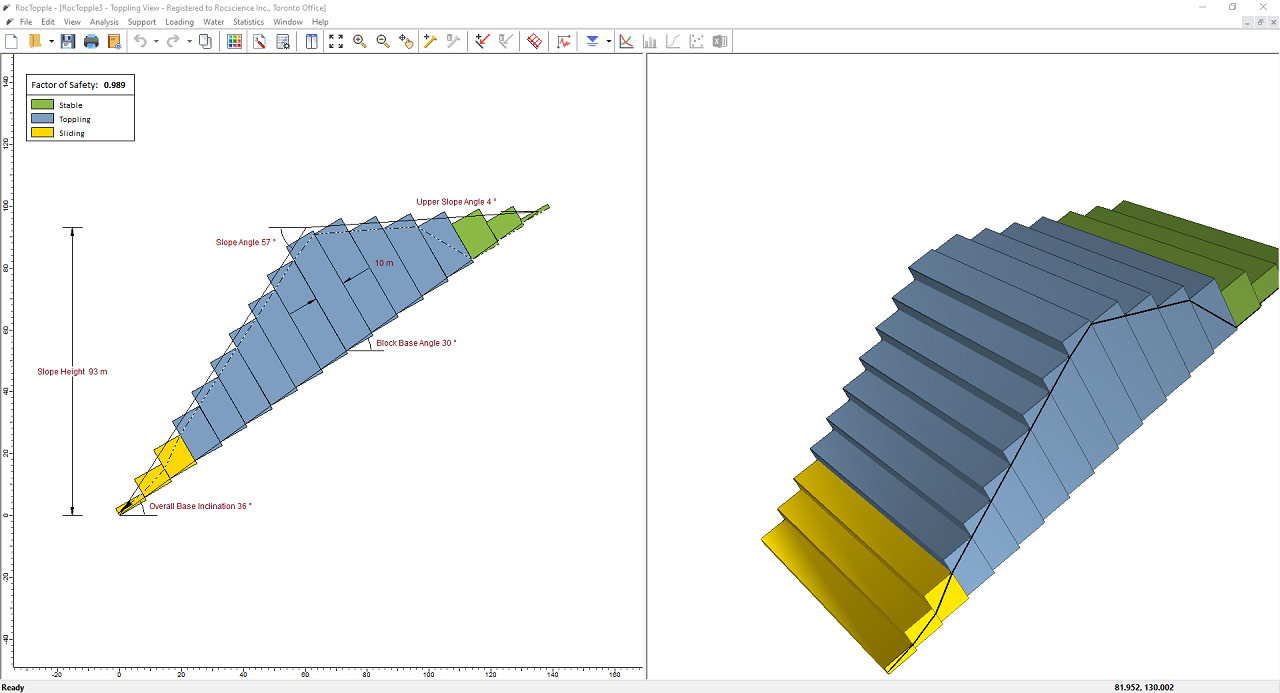If the RocTopple application window is not already maximized, maximize it now so that the full screen is available for viewing the model.

Before we are ready to run Automate Compute, we must first set up the model environment in the following dialogs:

1. Project Settings
2. Input Data
4. Water Pressure Options
NOTE: This RocTopple model file is meant to be used as a base file for automation. It contains the appropriate settings to be used for all automated cases and the engine for computation. It is not meant to be used for model creation and result viewing, as it does not save the model state for every case.

## 3.0 Project Settings

First, set the model Units, Analysis Type, and Block Shape.

1. Select Project Settings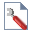from the Analysis menu to open the Project Settings dialog.
2. Set the Units = Metric, stress as kPa.
3. Set the Analysis Type = Probabilistic.
4. Set the Number of Samples = 5000.
5.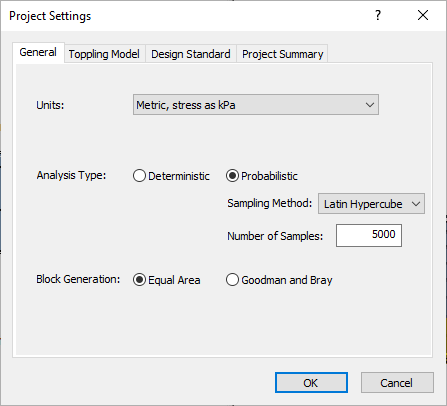6. In the Toppling Model tab, set the Toppling Model = Block Toppling.
7.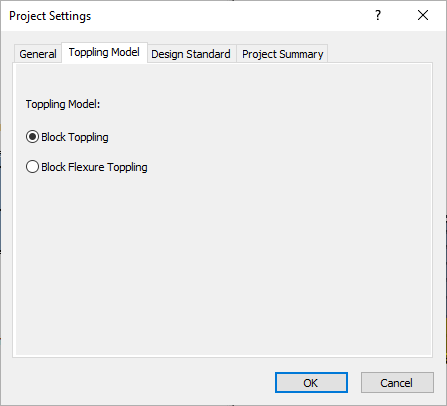8. Select OK to close the dialog.
NOTE: The above Project Settings will apply to all cases analyzed in Automate Compute. Each case being analyzed is Probabilistic (i.e. 5,000 sample toppling scenarios, as defined) in this case.

## 4.0 Input Data

Next, set the optional input data.

1. Select Input Datafrom the Analysis menu to open the Probabilistic Input Data dialog.
2. Navigate to the Geometry tab.
1. Set the Slope Angle = Normal.
2. Set the Slope Height = Normal.
3. Set the Rock Unit Weight = Normal.
3. Keep all other Statistical Distributions = None and check box controls in the default state. Keep in mind that the following are required input parameters that have to be specified later on in the Input Excel File:

• Slope Angle Mean, Standard Deviation, Relative Minimum, and Relative Maximum
• Slope Height Mean, Standard Deviation, Relative Minimum, and Relative Maximum
• Upper Slope Angle Mean
• Toppling Joints Spacing Mean
• Toppling Joints Dip Mean
• Overall Base Inclination Angle Mean
• Rock Unit Weight Mean, Standard Deviation, Relative Minimum, and Relative Maximum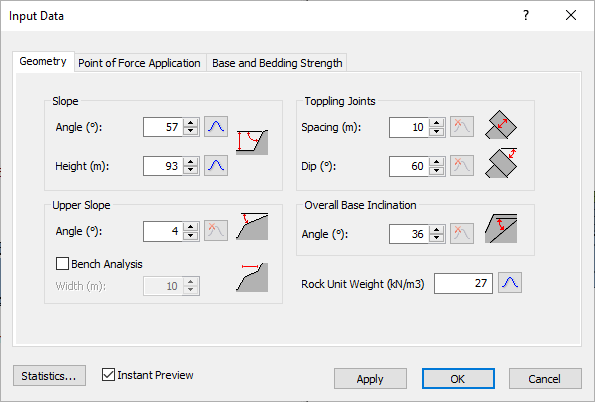4. Navigate to the Point of Force Application tab.
5. Keep all the Statistical Distributions = None. Keep in mind that the following are required input parameters that have to be specified later on in the Input Excel File:

• If Block Above is Sliding
• If Block Above is Shearing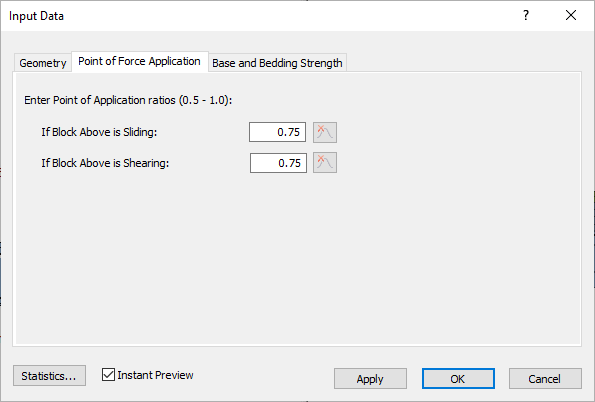6. Navigate to the Base and Bedding Strength tab.
1. Set Base Joints Shear Strength Model = Mohr-Coulomb.
2. Set the Base Joints Friction Angle = Normal.
3. Set Toppling Joints Shear Strength Model = Mohr-Coulomb.
4. Set the Toppling Joints Friction Angle = Normal.

Keep all other Statistical Distributions = None. Keep in mind that the following are required input parameters that have to be specified later on in the Input Excel File:

• Base Joints Friction Angle Mean, Standard Deviation, Relative Minimum, and Relative Maximum
• Base Joints Cohesion Mean
• Base Joints Tensile Strength Mean
• Toppling Joints Friction Angle Mean, Standard Deviation, Relative Minimum, and Relative Maximum
• Toppling Joints Cohesion Mean
• Toppling Joints Tensile Strength Mean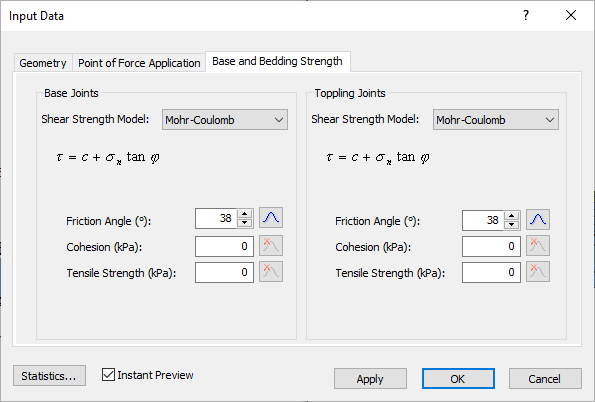7. Select OK to close the dialog.
NOTE: You can learn about RocTopple input data in detail in Overview of RocTopple Input Data.

## 5.0 Input Excel File

Now, let's take a look at the structure of the input Excel file.

1. Obtain the RocTopple Probabilistic Excel Template by selecting Analysis > Automate Compute > Probabilistic Excel Template from the menu.
2. A Read-Only Excel workbook titled RocTopple Automation Template_Probabilistic.xlsx will be automatically opened (if you have Excel installed on your local machine).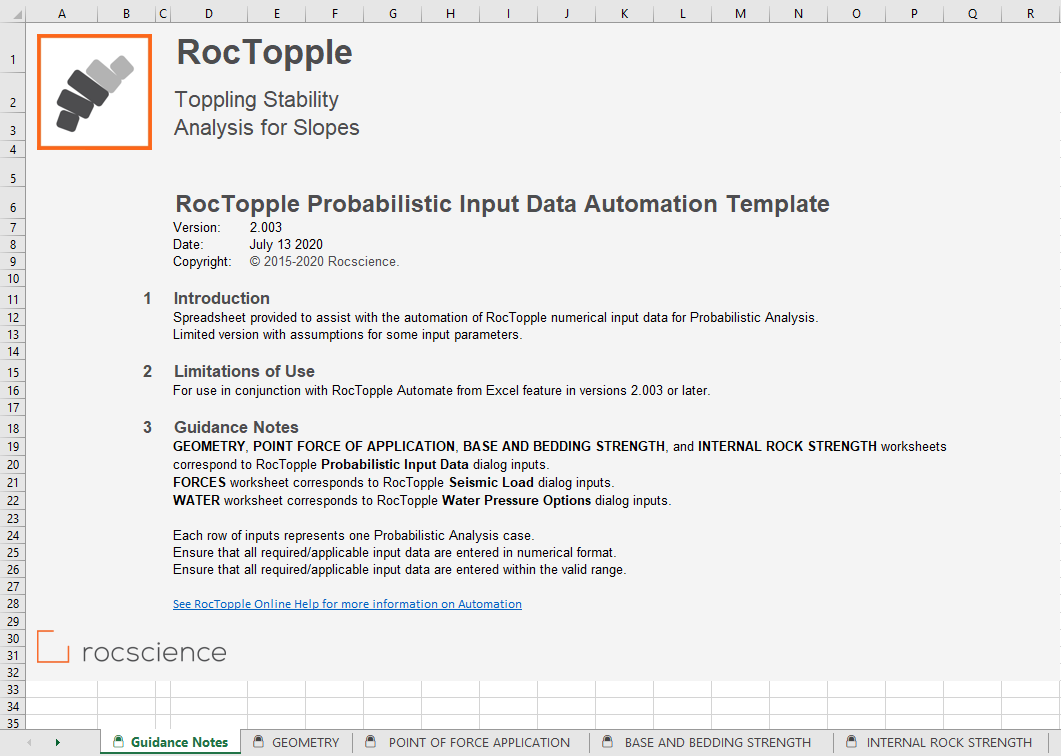The RocTopple Probabilistic Input Data Automation Template Excel workbook and worksheets are protected to prevent any accidental modification to the tab structure and headers. The input Excel template contains 6 worksheets for inputting numerical parameters for automation.

3. Create a folder called Tutorial 8 Automate Compute - Probabilistic.
4. Navigate to the GEOMETRY worksheet.
5. Under the Topple ID column, provide the names of the toppling cases. We will name them arbitrarily Topple A, Topple B, and Topple C. Each row in the input Excel file represents one Probabilistic Analysis case in RocTopple.
6. Enter the following for:
7.  Topple ID Slope Upper Slope Toppling Joints Overall Base Inclination Rock Unit Weight Angle Height Angle Spacing Dip Angle Mean Std. Dev. Rel. Min. Rel. Max. Mean Std. Dev. Rel. Min. Rel. Max. Mean Mean Mean Mean Mean Std. Dev. Rel. Min. Rel. Max. Topple A 52 2 6 6 84 3 9 9 27 10 50.5 45 25 1 3 3 Topple B 48 1.5 4.5 4.5 72 2.1 6.3 6.3 30 8 55 42 23 1.1 3.3 3.3 Topple C 59 2.8 8.4 8.4 88 3.5 10.5 10.5 25 5 52.5 48 26 1.5 4.5 4.5
NOTE: As set up previously in the RocTopple Probabilistic Input Data dialog, only the above input data are required since Bench Width and other Statistical Distributions are not applicable. Be aware of units set in RocTopple Project Settings when entering data into the input Excel file. The units should be the same for both!

The GEOMETRY worksheet should look like this: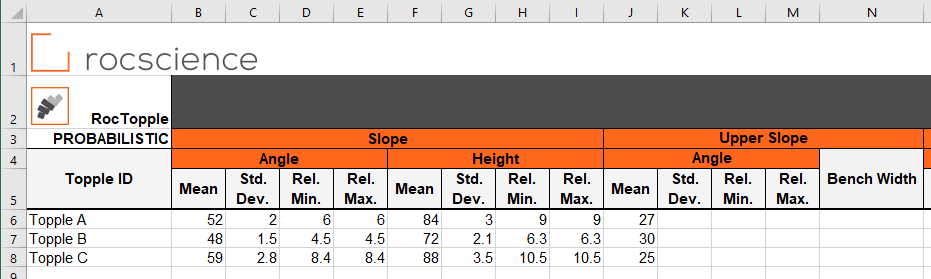8. Navigate to the POINT OF FORCE APPLICATION worksheet.
1. Under the Topple ID column, provide the names of the wedges (Topple A, Topple B, and Topple C).
2. Enter the following:
 Point of Force Application Ratios Topple ID If Block Above is Sliding If Block Above is Shearing Mean Mean Topple A 0.85 0.85 Topple B 0.78 0.79 Topple C 0.75 0.73
NOTE: As set up previously in the RocTopple Project Settings dialog, only the above input data are required since Flexural Bending parameters are not applicable.

The POINT OF FORCE APPLICATION worksheet should look like this: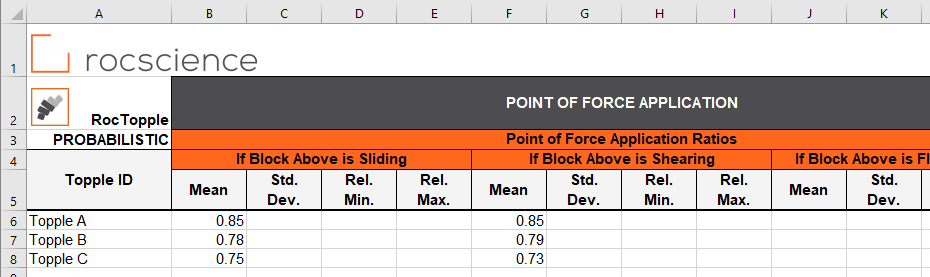9. Navigate to the BASE AND BEDDING STRENGTH worksheet.
1. Under the Topple ID column, provide the names of the wedges (Topple A, Topple B, and Topple C).
2. Enter the following:
 Base Joints Toppling Joints Topple ID C Phi Tensile Strength C Phi Tensile Strength Mean Mean Std. Dev. Rel. Min. Rel. Max. Mean Mean Mean Std. Dev. Rel. Min. Rel. Max. Mean Topple A 0.25 35 3 9 9 1 0.24 34.5 2.9 8.7 8.7 0 Topple B 0.27 40 3.2 9.6 9.6 1.5 0.23 36 3.1 9.3 9.3 1 Topple C 0.23 32 2.1 6.3 6.3 1.1 0.22 30.5 2.7 8.1 8.1 0.9
NOTE: As set up previously in the RocTopple Deterministic Input Data dialog, only the above input data are required since only Mohr-Coulomb parameters are applicable. Be aware of units set in RocTopple Project Settings when entering data into the input Excel file. The units should be the same for both!

The BASE AND BEDDING STRENGTH worksheet should look like this: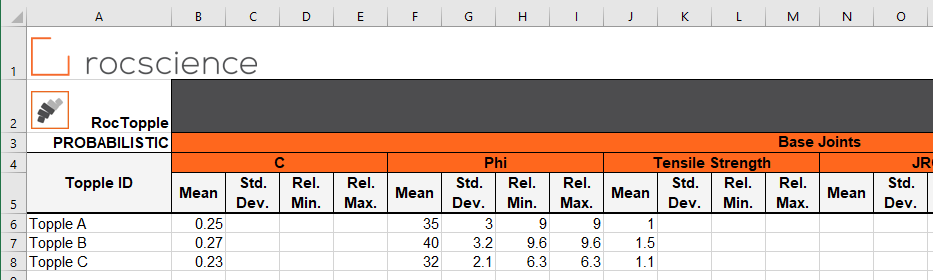We do not need to enter any data in the INTERNAL ROCK STRENGTH, FORCES, or WATER worksheets since those are not applicable.

10. Save the Excel file as Tutorial 8 Automate Compute - Probabilistic_inputs.xlsx to the Tutorial 8 Automate Compute - Probabilistic folder previously created.
NOTE: Since the RocTopple Automation Template_Probabilistic.xlsx file is Read-Only, you must rename and save in a new location.

We are now ready to automate computations in RocTopple by reading from the input Excel file.

## 6.0 Automate from Excel

The Automate Compute dialog allows you to add Excel input automation files to the queue, select a an output format, and compute the results. To open the dialog:

1. Select: Analysis > Automate Compute > Automate from Excel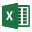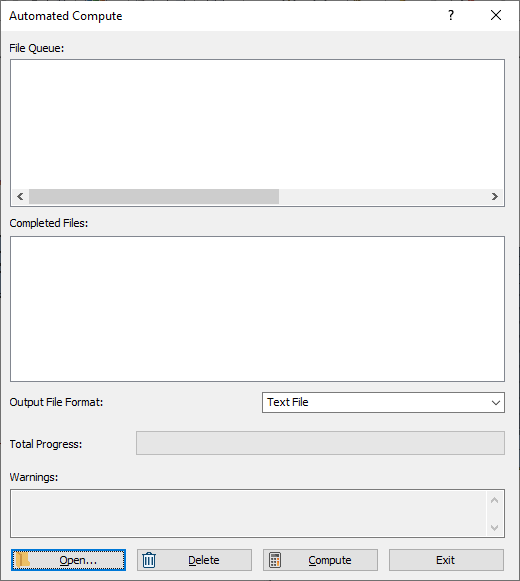### 6.1 ADD FILES TO FILE QUEUE

To add multiple files to the File Queue:

1. Select Automate from Excelfrom the Analysis menu to open the Automated Compute dialog.
2. Select the Open button to open a standard dialog to choose an Excel input file. Navigate to the Tutorial 8 Automate Compute - Probabilistic folder and select the Tutorial 08 Automate Compute - Probabilistic_inputs.xlsx file.

You will see the Excel input file appear under the File Queue list box.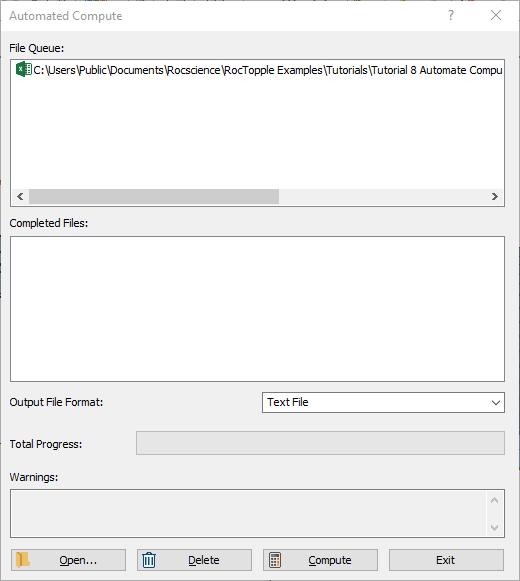## 7.0 Output Result Files

Results files are automatically generated and saved into the same directory as the input Excel file after Automated Compute is complete. Before we run Compute, first ensure that the Output File Format is set to the desired format. Users can select from either Text File or Excel format.

### 7.1 TEXT FILE FORMAT

While still in the Automated Compute dialog:

1. Set the Output File Format dropdown to Text File.
2. Select the Compute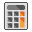button to start the automated computing process.
3. The Total Progress can be seen at the bottom of the dialog. When files have finished computing, they will appear in the Completed Files list box.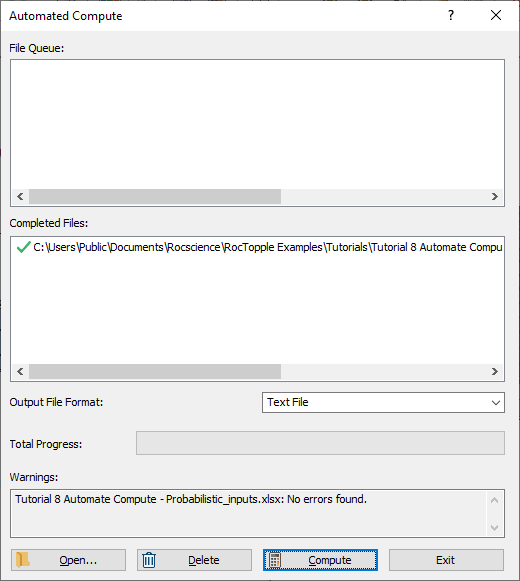4. When all files have finished computing, select Close to exit the dialog.

For each input Excel file case, a text file (.txt) containing all results data is generated..

For a Probabilistic Analysis (Tutorial 8 Automate Compute - Probabilistic.xlsx), the results data for each case are output to a separate text file since each case tends to contain hundreds or thousands of topple samples. Inside each text file, results for all probabilistic topple samples in the case are outputted following the header row. Each row of data represents one sample topple case. Each data type is separated by a comma (,) for easy parsing.

1. Take a look at the ResultsTopple A.txt result file.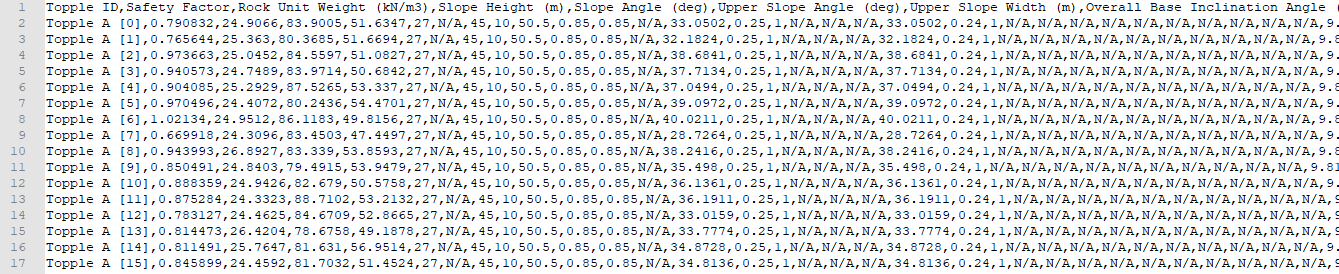Note that a total of 3 files with the same format (i.e., ResultsTopple A.txt, ResultsTopple B.txt, ..., ResultsTopple C.txt ) have been created; one file per case.

### 7.2 EXCEL FILE FORMAT

Now, we will do the same as before, but this time we will generate the results as an Excel output file format.

1. Select Automate from Excelfrom the Analysis menu to open the Automated Compute dialog.
2. Select the Open button to open a standard dialog to choose an Excel input file. Navigate to the Tutorial 8 Automate Compute - Probabilistic folder and select the Tutorial 8 Automate Compute - Probabilistic.xlsx file.
3. You will see the Excel input file appear under the File Queue list box.

4. Set the Output File Format dropdown to Excel.
5.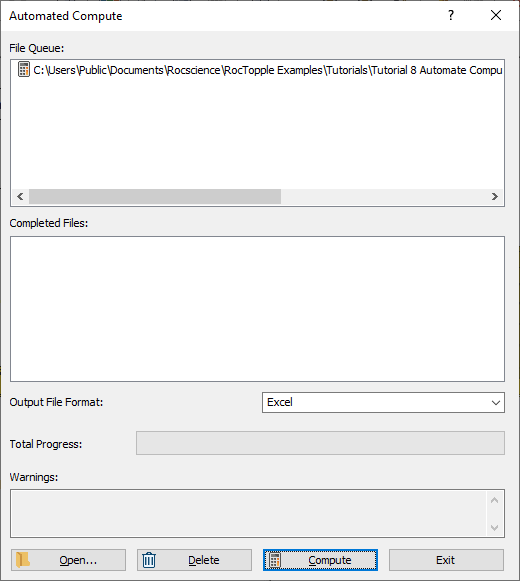6. Select the Computebutton to start the automated computing process.
7. When all files have finished computing, select Close to exit the dialog.

For each input Excel file case, an Excel file (.xlsx) containing all results data is generated..

For a Probabilistic Analysis (Tutorial 8 Automate Compute - Probabilistic.xlsx), the results data for each case are output to a separate Excel file since each case tends to contain hundreds or thousands of topple samples. Inside each Excel file, results for all probabilistic topple samples in the case are outputted following the header row. Each row of data represents one sample topple case. Each data type is separated by column.

1. Take a look at the ResultsTopple A.xlsx result file.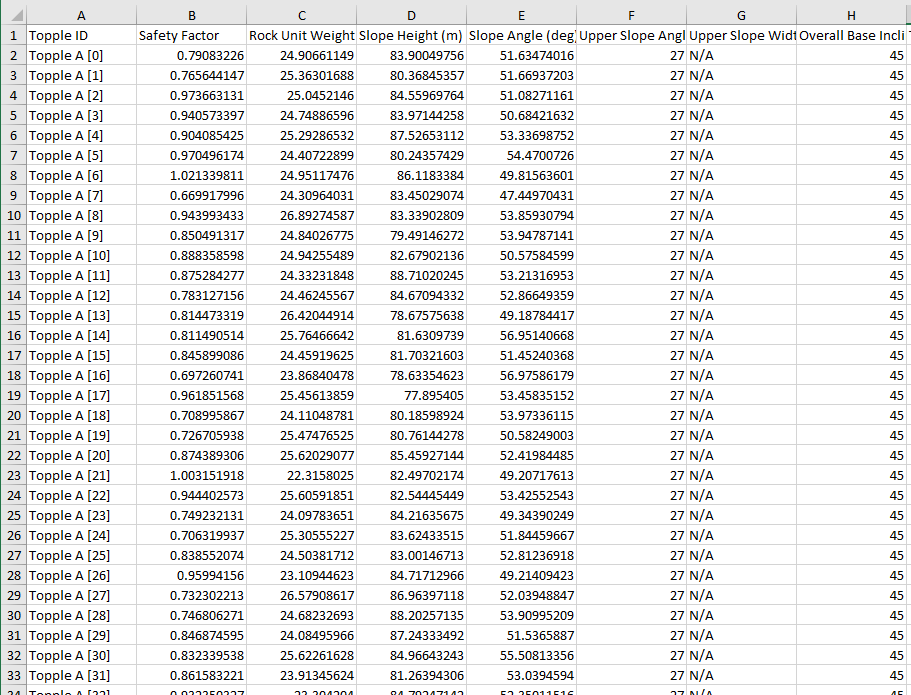Note that a total of 3 files with the same format (ResultsTopple A.xlsx, ResultsTopple B.xlsx, ..., ResultsTopple C.xlsx ) have been created; one file per case.

## 8.0 Error Log

As you may have noticed, an Error Log is also generated for each input Excel file. For each input Excel file, an Error Log text file (Automate Compute Error Log.txt) is generated, and saved into the same directory as the output file directories. Always check that the Error Log reports "No errors found" before post-processing the raw results data. If any errors do occur as a result of missing (i.e., blank cells) or invalid inputs, the Error Log will indicate which worksheet(s) and cell(s) are problematic.

This concludes the tutorial.